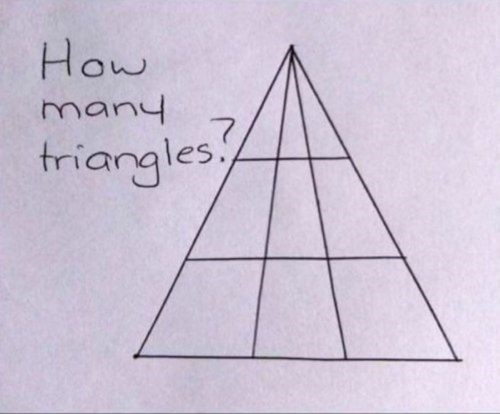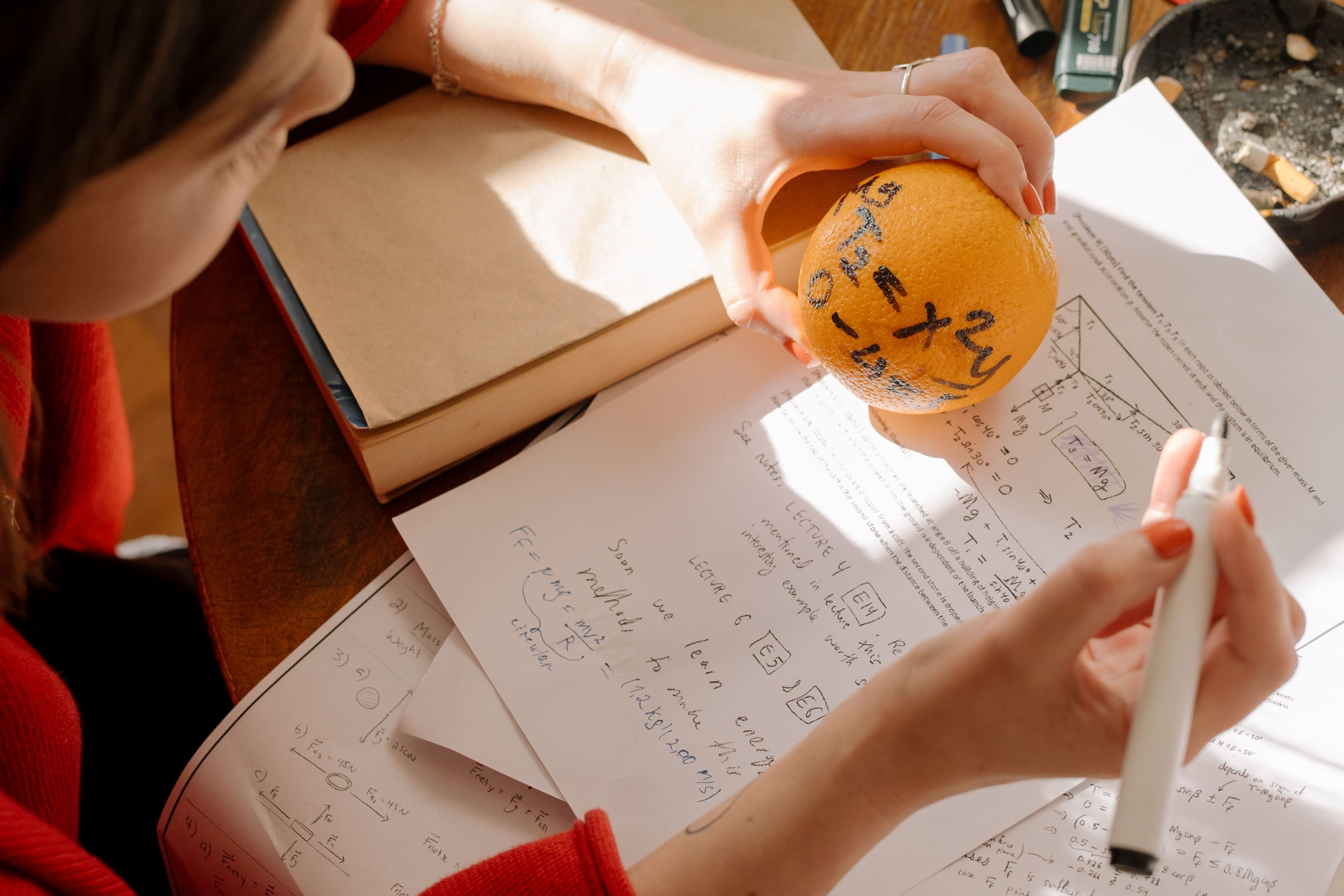# 20 Funny Math Questions with Answers

If you approach math with the right attitude, it can be fun. You will encounter funny math questions that jog your mind and come with the most interesting answers. Most of the questions test your logic. Others come with illusions that result in the wrong answers. The funny math answers are equally interesting.

Here are tricky math equations and questions that will blow your mind.

1. I am an odd number. Take away one letter, I will become even. Which number am I?

Answer: Seven. Seven is an odd number. If you remove ‘S’ in the beginning, you will be
left with ‘even.’

1. How do you add 8s to get 1000?

1. John is 54 and his father is 80. How many years ago was John’s father 3 times John’s age?

Answer: 41 years ago. John was 13 while his father was 39 years old.

1. Here is an example of funny math questions that test your logic. There are 6 bananas in a basket. How do you share them among 6 children so that one banana remains in the basket yet each child gets one banana?

Answer: Give five children a banana each, then hand over the remaining banana to the last kid while it is still inside the basket.

1. Two boys were born at the same time, to the same mother, in the same year, the same month, and day, yet they are not twins. How?

Answer: they are triplets, meaning there is a third kid.

1. I was 25 the day before yesterday. I will be 28 next year. It is only possible one day a year. On what day is my birthday?

1. Use 4 sevens (7) to get 100.

1. Here is another interesting math problem to jog your logic. In the following series, which number comes next 36, 34, 30, 28, 24, …

1. Which three numbers will give the same answer whether they are multiplied or added together?

1. Here is a tricky Maths question that does not require any calculation. Which word looks the same backward as well as upside down?

1. Finish this equation

1=4, 2=16,3=64, 4= ?

1. Here is part of the funny maths tests that will push your logic to the limits.1. A patch of lily doubles in size each day. If it takes 48 days to cover the entire pond, how long would it take to cover half the pond?

1. How many feet are in a mile?

1. What do you get when you divide 1.92 by 3?

1. A warehouse has 85 people. Some of them have turned into zombies. Others are still alive. The ratio of humans to zombies is 3:2. How many zombies are in the warehouse?

1. A ship at the port hangs a ladder on the outside. The ladder is 200 cm long. Each rung is 20 cm away from the next. The bottom run is touching the water. If the tide rises at 10cm per hr, how long will it take for water to get to the 5th rung?

Answer: It will not. The ship rises as the tide rises.

1. A figure has three-digit numbers. The third digit is three times less than the second digit. The second digit is four times more than the first digit. Which number is it?